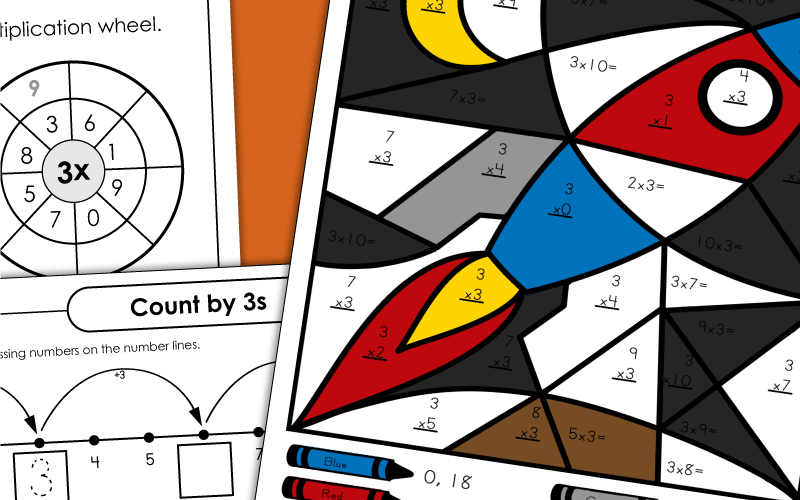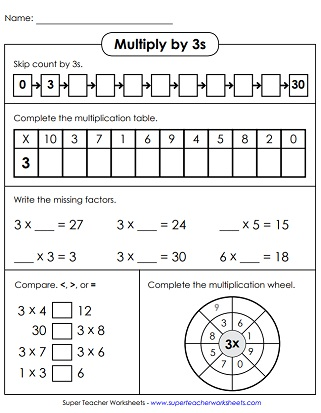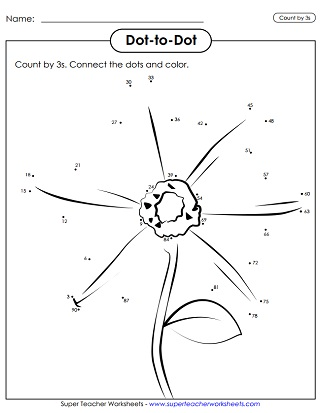# Multiplication by 3s

These printables focus specifically on the 3s multiplication facts. Browse through our collection of worksheets, task cards, foldable crafts, flash cards, and more.## Multiply by3s Only

This page has a 3s multiplication wheel, comparing questions, skip counting, and a table for students to fill in.
This mystery picture has all of the 3s times tables. Answer the math facts and color.
Multiply by 3 and write the answers. Then color the picture according to the key at the bottom of the page. Finished picture is a sailboat.
Task cards work well for peer practice, document cameras, classroom scavenger hunts, exit slips, and class games. Each card has an array, a repeated addition expression, a groups model, or a number family triangle.
Students will practice their multiplication facts with the numbers 3 and 4 with this puzzle activity.
Multiply by 3s to fill in all the bubbles on the multiplication caterpillar.
Create an origami cootie catcher (aka "fortune teller"). Kids can use it to learn and review basic facts.
Print these flash cards on card stock. As student practice, they can use the sorting mat to divide the cards into two groups- ones they have mastered and ones they have not.
Use this cool slider craft to practice multiplication facts. For best results, print on card stock.

## Skip Count by 3s

Determine the number of fish, flowers, crayons, and snails by counting by 3s. Up to 27, which coincides with 3x9.
Count by 3s up to 36. These numbers coincide with multiplication facts up to 3x12.
Skip count by 3s to make a beautiful flower picture. Start at number 3 and keep drawing until you get all the way up to 90.
Count by 3s and write the numbers on the penguins' bellies. Numbers up to 78.
Count by 3s and write the numbers on the balloons. Numbers to 72.
Count by 3s on the hundreds chart. Color every 3rd number. Then tell if the numbers are odd, even, or both.

## All Facts0s through 3s

This worksheet has 41 multiplication facts, for 0s, 1s, 2s, and 3s only. Facts through 10, up to 3x10.
Answer over 40 multiplication facts, with factors of 0, 1, 2, and 3. Facts through 12, up to 3x12.
This 50-question quiz has all multiplication facts for 0s, 1s, 2,s and 3s. Facts through 12, up to 3x12.
Multiplication Basic Facts: 0-10

Here you'll find lots more multiplication worksheets, games, and activities for all basic facts up to 10x10.

Multiplication/Division
Fact Families

This page has lots of worksheets with multiplication and division fact families.

Multiplication by 4s

These worksheets focus specifically on the 4s times tables.

Multiplication by 5s

Here are the activities for learning multiplication facts from 5x0 up to 5x12.

Multiplication by 6s

Multiplication by 7s

Basic facts with factors of 7 are the focus on these printable learning resources.

Multiplication by 8s

Practice multiplying by the number 8. Includes mystery pictures, skip counting lessons, and more.

Multiplication by 9s

## Sample Worksheet ImagesMy Account
Site Information